# Roots and coefficient

In the equation 2x ^ 2 + bx-9 = 0 is one root x1 = -3/2. Determine the second root and the coefficient b.

b =  -3
x2 =  3

### Step-by-step explanation:

Our quadratic equation calculator calculates it.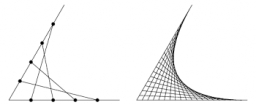Did you find an error or inaccuracy? Feel free to write us. Thank you!Tips to related online calculators
Looking for help with calculating roots of a quadratic equation?
Do you have a linear equation or system of equations and looking for its solution? Or do you have a quadratic equation?

## Related math problems and questions:

• Roots count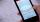Substitute the numbers/0,1,2,3/into the equation as x: (x - 1) (x - 3) (x + 1) = 0 Which of them is its solution? Is there another number that solves this equation?
• EquationEquation -2x2+bx -82 =0 has one root x1 = -8. Determine the coefficient b and the second root x2.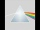Please determine the solvability conditions of the equation, solve the equation and perform the test: x divided by x squared minus 2x plus1 the whole minus x + 3 divided by x squared minus 1 this is equal to 0: x/(x2-2x+1) - (x+3)/( x2-1) = 0Determine the numbers b, c that the numbers x1 = -1 and x2 = 3 were roots of quadratic equation: -3x 2 + b x + c = 0
• Equation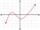Eequation f(x) = 0 has roots x1 = 64, x2 = 100, x3 = 25, x4 = 49. How many roots have equation f(x2) = 0 ?
• Sphere from tree pointsEquation of sphere with three point (a,0,0), (0, a,0), (0,0, a) and center lies on plane x+y+z=a
• Square root 2If the square root of 3m2 +22 and -x = 0, and x=7, what is m?Quadratic equation 7x2+bx+c=0 has roots x1 = 67 and x2 = -84. Calculate the coefficients b and c.
• Sphere equationObtain the equation of sphere its centre on the line 3x+2z=0=4x-5y and passes through the points (0,-2,-4) and (2,-1,1).
• Trapezoid 15Area of trapezoid is 266. What value is x if bases b1 is 2x-3, b2 is 2x+1 and height h is x+4
• CoefficientDetermine the coefficient of this sequence: 7.2; 2.4; 0.8
• Find the 20Find the product and the sum of the roots of x2 + 3x - 9 = 0
• CoordinatesDetermine the coordinates of the vertices and the content of the parallelogram, the two sides of which lie on the lines 8x + 3y + 1 = 0, 2x + y-1 = 0 and the diagonal on the line 3x + 2y + 3 = 0
• VCP equationSolve the following equation with variations, combinations and permutations: 4 V(2,x)-3 C(2,x+ 1) - x P(2) = 0
• Function 3Function f(x)=a(x-r)(x-s) the graph of the function has x- intercept at (-4, 0) and (2, 0) and passes through the point (-2,-8). Find constant a, r, s.
• Profit, revenue, cost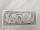Profit, P(x), is the difference between revenue, R(x), and cost, C(x), so P(x) = R(x) - C(x). Which expression represents P(x), if R(x) = 3x3 + 2x - 1 and C(x) = x4 - x2 + 2x + 3?Find the roots of the quadratic equation: 3x2-4x + (-4) = 0.